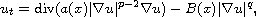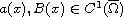Electron. J. Differential Equations, Vol. 2017 (2017), No. 311, pp. 1-15.

### Existence of solutions to an evolution p-Laplacian equation with a nonlinear gradient term Huashui Zhan, Zhaosheng Feng

Abstract:
We study the evolution p-Laplacian equation with the nonlinear gradient termwhere, p>1 and p>q>0. When a(x)>0 and B(x)>0, the uniqueness of weak solution to this equation may not be true. In this study, under the assumptions that the diffusion coefficient a(x) and the damping coefficient B(x) are degenerate on the boundary, we explore not only the existence of weak solution, but also the uniqueness of weak solutions without any boundary value condition.

Submitted April 9, 2017. Published December 31, 2017.
Math Subject Classifications: 35L65, 35K85, 35R35.
Key Words: Evolution p-Laplacian equation; weak solution; uniqueness; boundary value condition.

Show me the PDF file (279 KB), TEX file for this article.Huashui Zhan School of Applied Mathematics Xiamen University of Technology Xiamen, Fujian 361024, China email: huashuizhan@163.com Zhaosheng Feng Department of Mathematics University of Texas-Rio Grande Valley Edinburg, TX 78539, USA email: zhaosheng.feng@utrgv.edu